# Using Quasi-Isothermal MDSC for Heat Capacity Measurements

The energy needed to increase the temperature of a material is measured as heat capacity, which is a structure sensitive property. Traditional differential scanning calorimetry (DSC) can readily measure heat capacity between -100 and 725°C. The measurement is made by heating a test sample at a fixed heating rate over a specified temperature range. The resulting heat flow response, normalized for sample mass and heating rate, is directly proportional to specific heat via: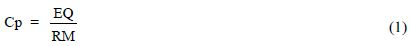Where, Cp is the specific heat capacity, E is the DSC calibration factor, Q is the heat flow, R is the heating rate and M is the sample mass. Modulated DSC™ (MDSC) is another method of measuring heat capacity with added benefits over traditional DSC method. With MDSC, non-reproducible experimental artifacts can be separated from the measurement, thereby providing better accuracy and precision. In addition, Cp results can be obtained at low heating rates and even under "isothermal" conditions. This article discusses Cp measurement under isothermal conditions.

## Theory

Conventional DSC involves determining the difference in heat flow between a blank (empty pan) run and a sample run under identical conditions (including same heating rate) to calculate Cp. For that approach, Cp can be expressed as: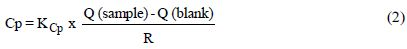Where KCp is the Cp cell calibration constant

It is also possible to calculate Cp through comparison of the difference in heat flow between two runs on an identical sample, but at two different heating rates (R1 and R2). Equation 1 can be expressed for that approach as follows: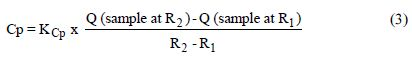Where R2 > R1

Typical results for sapphire are presented in Curves 1 and 2 in Figure 1. Sapphire run at a faster heating rate is represented in Curve 3 in Figure 1. The Cp values at 50°C estimated from those curves utilizing Equations 2 and 3 are 14.7 and 14.5mJ/C, respectively. In the MDSC method, the modulated heat flow amplitude is divided by the modulated heating rate amplitude to determine Cp.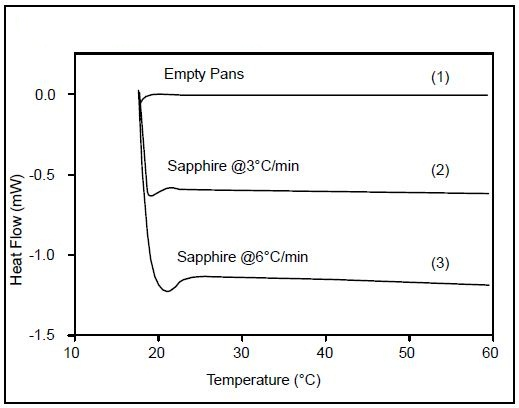Figure 1. Sapphire heat capacity - conventional DSC

The curves in Figure 2 demonstrate the validity of the MDSC method, where the MDSC conditions are selected in such a way that the modulated heating rate changes between two heating rates which are the same as those selected for curves 2 and 3 in Figure 1. The resultant heat flows at the extremes of the modulated heat flow curve directly relate to curves 2 and 3 from Figure 1, thus validating Equation 3.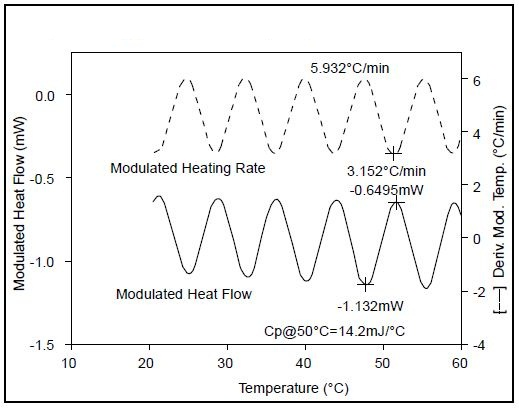Figure 2. Sapphire heat capacity - modulated DSC

However, multiple conventional DSC experiments need to be performed to get reliable results compared to a single experiment in the case of modulated DSC. In addition, in MDSC, the modulated heating rate amplitude and the resultant modulated heat flow amplitude rely on the underlying heating rate, modulation amplitude and period. Therefore, finite values can be obtained for the modulated heating rate, the modulated heat flow, and therefore Cp when the underlying heating rate is zero under "quasi-isothermal" conditions.

## Applications of MDSC Quasi-Isothermal Measurements

### Improved Cp Measurement in Metals

Most metals have low heat capacity values relative to other materials such as polymers. Hence, the accuracy of the results will be significantly affected even for minor experimental variations. Table 1 shows the ability of MDSC™ quasi-isothermal measurements to provide accurate results over a broad temperature range even with low heat capacity metals.

Table 1. Copper heat capacity - Modulated DSC

Temp. (°C) Cp (MDSC) (J/g/°C) Cp (Liter.) (J/g/°C)
-154.3 0.2462 0.2812
-102.4 0.3116 0.3401
-51.5 0.3432 0.3656
-1.1 0.3639 0.3783
49.2 0.3737 0.3877
99.3 0.3784 0.3971
199.4 0.3863 0.4128
249.4 0.3977 0.4187
299.5 0.4175 0.4284

## Increased Tg Accuracy and Precision

DSC measures glass transitions in polymers based on the change in heat capacity that takes place. The size of this change, and hence the ability to detect the glass transition, increases as the heating or cooling rate increases. However, the heating/cooling rate not only affects the sensitivity but also the temperature and shape of the transition as shown by the solid curves in Figure 3. Conversely, steady-state glass transition shape and temperature can be obtained with MDSC as shown by the dashed curve in Figure 3.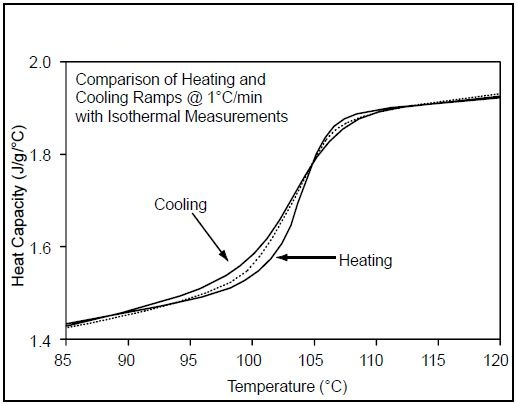Figure 3. Polystyrene glass transition

## Heat Capacity During Cure

Conventional DSC cannot perform heat capacity measurements during the isothermal cure of thermosetting polymers due to the absence of heating rate and the simultaneous occurrence of the exothermic heat flow associated with curing. However, the nonreversing (kinetic) curing process is separated from Cp by MDSC, allowing the quantification of both the values (Figure 4).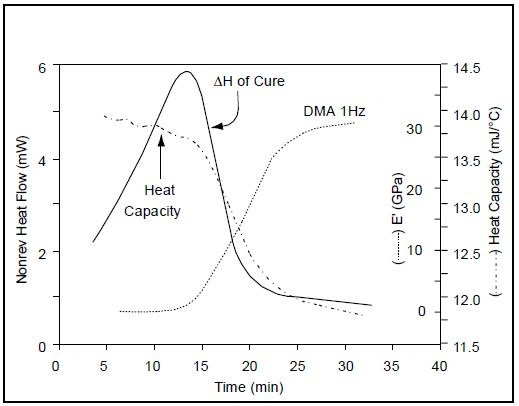Figure 4. Isothermal epoxy cure

## Heat Capacity During Cold Crystallization and Melting

Figure 5 presents the results of heat capacity changes during cold crystallization, whereas Figures 6 and 7 present the results of heat capacity changes during melting. Both these results illustrate the ability of MDSC to provide additional information that is not possible with conventional DSC.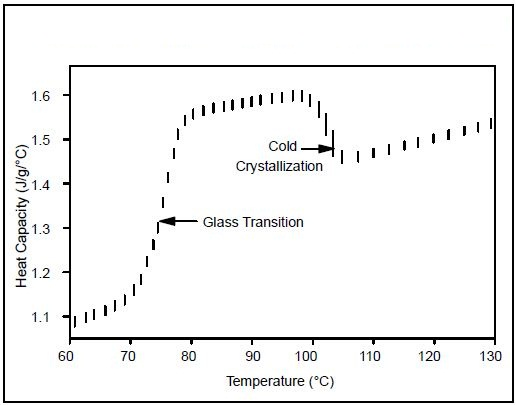Figure 5. Heat capacity change at cold crystallization

Heat capacity increases initially during cold crystallization due to increased molecular mobility above Tg, but declines due to the occurrence of the rearrangement to a more stable crystalline form. The separation of Cp from the exothermic kinetic crystallization process and the improved reproducibility of the quasi-isothermal measurement are the key advantages of the MDSC method.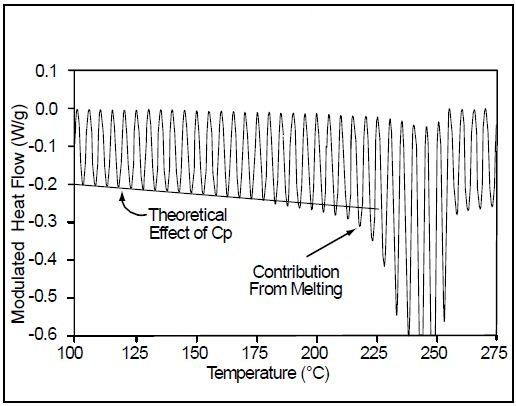Figure 6. Slow cooled PET

During melting, it is difficult to perform Cp measurement using MDSC with an underlying heating rate higher than zero due to the contribution of two "apparently reversing" phenomena (Cp and melting) to the modulated heat flow amplitude, creating an artificially high Cp value.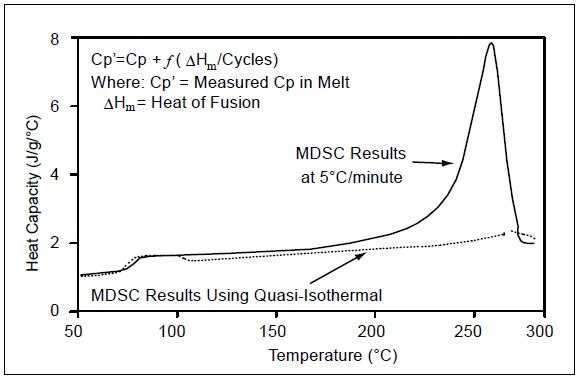Figure 7. Cp in melt region by MDSC

The heat of melting is effectively extended over a very large number of modulations under quasi-isothermal conditions, minimizing the effect attributable to melting and getting Cp trends in line with theoretical predictions. However, this latter measurement is relatively new and therefore it is essential to carry out more research on this measurement in order to identify its practical limits.This information has been sourced, reviewed and adapted from materials provided by TA Instruments.

## Citations

• APA

TA Instruments. (2020, April 28). Using Quasi-Isothermal MDSC for Heat Capacity Measurements. AZoM. Retrieved on September 24, 2020 from https://www.azom.com/article.aspx?ArticleID=12119.

• MLA

TA Instruments. "Using Quasi-Isothermal MDSC for Heat Capacity Measurements". AZoM. 24 September 2020. <https://www.azom.com/article.aspx?ArticleID=12119>.

• Chicago

TA Instruments. "Using Quasi-Isothermal MDSC for Heat Capacity Measurements". AZoM. https://www.azom.com/article.aspx?ArticleID=12119. (accessed September 24, 2020).

• Harvard

TA Instruments. 2020. Using Quasi-Isothermal MDSC for Heat Capacity Measurements. AZoM, viewed 24 September 2020, https://www.azom.com/article.aspx?ArticleID=12119.